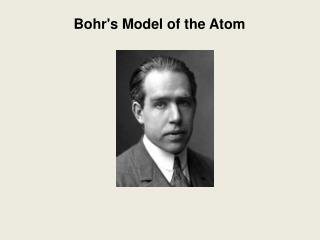DownloadDownload PresentationBohr's Model of the Atom

# Bohr's Model of the Atom

Télécharger la présentation## Bohr's Model of the Atom

- - - - - - - - - - - - - - - - - - - - - - - - - - - E N D - - - - - - - - - - - - - - - - - - - - - - - - - - -
##### Presentation Transcript

1. Bohr's Model of the Atom

2. Bohr's Model of the Atom Niels Bohr (1913):

3. Bohr's Model of the Atom Niels Bohr (1913): -studied the light produced when atoms were excited by heat or electricity

4. Bohr's Model of the Atom Niels Bohr (1913): -studied the light produced when atoms were excited by heat or electricity

5. Bohr's Model of the Atom Niels Bohr (1913): -studied the light produced when atoms were excited by heat or electricity Rutherford's model couldn't explain why unique colours were obtained by atoms of different elements

6. Bohr's Model of the Atom Niels Bohr (1913): -studied the light produced when atoms were excited by heat or electricity Rutherford's model couldn't explain why unique colours were obtained by atoms of different elements Bohr proposed that electrons are in orbits & when excited jump to a higher orbit. When they fall back to the original they give off light

7. Bohr's Model of the Atom Bohr's model: -electrons orbit the nucleus like planets orbit the sun

8. Bohr's Model of the Atom Bohr's model: -electrons orbit the nucleus like planets orbit the sun -each orbit can hold a specific maximum number of electrons

9. Bohr's Model of the Atom Bohr's model: -electrons orbit the nucleus like planets orbit the sun -each orbit can hold a specific maximum number of electrons

10. Bohr's Model of the Atom Bohr's model: -electrons orbit the nucleus like planets orbit the sun -each orbit can hold a specific maximum number of electrons -electrons fill orbits closest to the nucleus first.

11. Bohr's Model of the Atom e.g. fluorine: #P = #e- = #N =

12. Bohr's Model of the Atom e.g. fluorine: #P = atomic # = 9 #e- = #N =

13. Bohr's Model of the Atom e.g. fluorine: #P = 9 #e- = # P = 9 #N =

14. Bohr's Model of the Atom e.g. fluorine: #P = 9 #e- = 9 #N = atomic mass - # P = 10

15. Bohr's Model of the Atom e.g. fluorine: #P = 9 #e- = 9 #N = 10 draw the nucleus with protons & neutrons 9P 10N

16. Bohr's Model of the Atom e.g. fluorine: #P = 9 #e- = 9 #N = 10 how many electrons can fit in the first orbit? 9P 10N

17. Bohr's Model of the Atom e.g. fluorine: #P = 9 #e- = 9 #N = 10 how many electrons can fit in the first orbit? 2 9P 10N

18. Bohr's Model of the Atom e.g. fluorine: #P = 9 #e- = 9 #N = 10 how many electrons are left? 9P 10N

19. Bohr's Model of the Atom e.g. fluorine: #P = 9 #e- = 9 #N = 10 how many electrons are left? 7 9P 10N

20. Bohr's Model of the Atom e.g. fluorine: #P = 9 #e- = 9 #N = 10 how many electrons are left? 7 how many electrons fit in the second orbit? 9P 10N

21. Bohr's Model of the Atom e.g. fluorine: #P = 9 #e- = 9 #N = 10 how many electrons are left? 7 how many electrons fit in the second orbit? 8 9P 10N

22. Bohr's Model of the Atom e.g. fluorine: #P = 9 #e- = 9 #N = 10 9P 10N

23. Bohr's Model of the Atom try these: hydrogen boron magnesium

24. Bohr's Model of the Atom try these: hydrogen 1P 0N

25. Bohr's Model of the Atom try these: boron 5P 6N

26. Bohr's Model of the Atom try these: magnesium 12P 12N